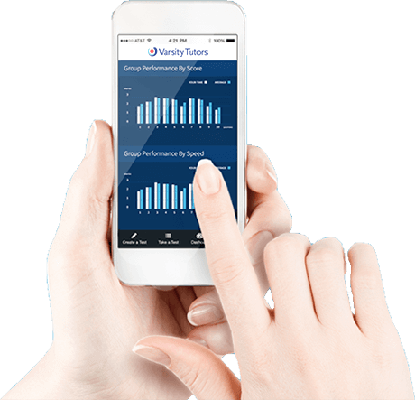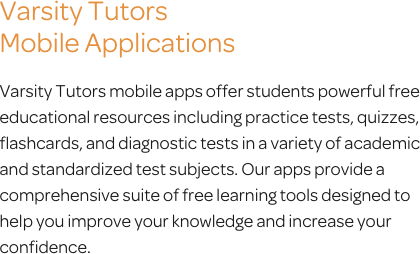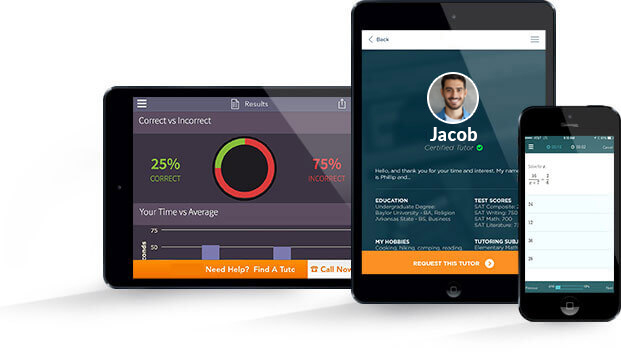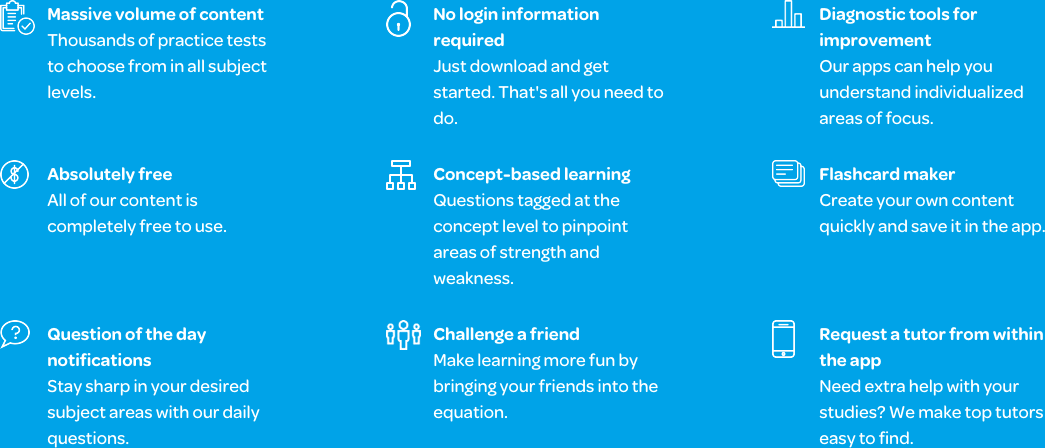# Varsity Tutors Pre-Algebra mobile app### The Varsity Tutors Pre-Algebra Mobile App

Prior to learning algebra, geometry, trigonometry, or calculus, your child must fully understand the elements taught in pre-algebra. This class can set them up for success in their upper-level math endeavors. The free Varsity Tutors Pre-Algebra app for iPhone, iPad, and Android devices thoroughly explain these math fundamentals. The app can help your student work toward the knowledge they need for better grades and gain a deeper understanding of essential mathematical concepts.

Pre-algebra is a subject generally taught in middle school. As the educational landscape gets more competitive, however, students are being exposed to these more advanced concepts at a younger age. When your student takes pre-algebra, they will learn how numbers work. They will learn to understand whole numbers, prime numbers, mixed numbers, real numbers, and many other concepts of number theory. They will be instructed in how to manipulate the types of numbers that they will need to know in Algebra 1. They will also be taught the properties of numbers, such as the associative, commutative, distributive, additive, and multiplicative properties.

After the basic number concepts are mastered, your student will learn about other important concepts, like absolute value. Your child will review addition, subtraction, multiplication, and division of whole numbers, fractions, and decimals. They will also begin mixing negative and positive numbers into these types of calculations.

Near the end of the course, basic one- and two-step algebraic equations will be introduced. Pre-algebra is the first step into the complex algebraic equations your student may perform in the future. Additionally, they will learn how to graph -- another essential math skill that your child will use for years to come.

If your son or daughter is one of the many students who do not have an easy time with math, studying can seem like a real struggle. With the Varsity Tutors Pre-Algebra app for iOS or Android devices, they will have a great number of practice resources that can help to streamline their review.

Have your child begin by taking one of the full-length, timed practice tests to see where their strengths lie, and where they might need more help. The results will help you to build a custom pre-algebra study plan for your child. For example, if they’re having trouble distinguishing between a rational and irrational number, Learn by Concept can help your child master that skill. Learn by Concept provides practice problems with comprehensive explanations that show exactly how the correct answer is reached.

If your student is looking for quick study sessions throughout the day, they can use the app’s flashcard section. They can use the wealth of cards already available on the app, or create a custom set with the Flashcard Maker. You and your child can use your own audio, photos, and text to personalize a set of flashcards.

If your child needs a little help with pre-algebra, try the free Varsity Tutors Pre-Algebra app. It’s a portable, comprehensive resource that enables your child to review anytime, anywhere. Download it from iTunes or the Google Play Store today!If you have a middle school student, they will probably need to complete a course in pre-algebra. This course is usually taught in either sixth or seventh grade, and is designed to introduce the basic concepts that will prepare them for more advanced algebra courses. As your child makes their way through this course, they will begin to get experience with the concept of abstract mathematics.

In pre-algebra, topics include learning other types of numbers, like integers, decimals, negative numbers, and fractions. Additionally, your child will learn about basic powers and roots, how to solve basic equations, and will develop an understanding of manipulated variables. While Common CORE curriculum varies from state to state, pre-algebra examinations should include the aforementioned material.

As your child gets started in pre-algebra, they will get an introduction to negative numbers. They will learn about what happens when you add and subtract negative numbers, and about the sequential order of negative numbers on the number line. After being familiarized with the basic concepts of negative numbers, your child will be required to put these concepts into action. By doing this, they will learn about what happens when you add and subtract two negative numbers, as well as what happens when you do the same actions with one negative and one positive number. Furthermore, they will be required to learn the rules of multiplication and division of negative numbers, as well as the concept of absolute value.

Understanding factors and multiples are important concepts that will aid your child as they progress through pre-algebra. In this area, they will learn about divisibility, and which numbers are divisible by other numbers. They will also learn about which factors make up various numbers, and how to find these factors. They will be exposed to prime numbers, and how they can’t have a positive divisor aside from the number one, and will learn how to use prime factorization to determine a set of prime numbers.

Rational numbers are an important component of algebraic mathematics. To become familiar with this concept, your child will be required to explore two types of numbers: decimals and fractions. By virtue of spending money and understanding the concept of dollars and cents, they may have a good understanding of the decimal system. In pre-algebra, however, they will need to know how to add, subtract, multiply, and divide decimals. Moreover, they will learn how to convert decimals to fractions and vice versa, as well as how to turn both decimals and fractions into a percentage. To better understand these concepts, you can practice with your child by applying them in a real-world setting. Try having them calculate the percentage of a tip for a restaurant bill, for instance.

Moving into fractions, you and your child can practice using visual activities in order to gain an understanding. Imagine a pizza that is cut into eight pieces. Each slice is considered one-eighth of a pizza. After developing a working knowledge of fractions, your child will learn how to incorporate them into math problems. Furthermore, they will learn about improper fractions and mixed numbers, how you can convert one to the other, and how you multiply and divide improper fractions and mixed numbers.

As pre-algebra progresses, your child will have the chance to get familiar with the concepts of proportions and ratios. Learning about ratios can be easy when applied in realistic terms. Have your child try and imagine the ratio of boys-to-girls in a classroom, or the student-to-teacher ratio in their favorite class. Along with learning about how a ratio is a comparison of two things, they will also learn how to incorporate them into mathematic problems. Proportions are similar to ratios, except that they are a pair of ratios which are equal to one another. For example, if you are expected to eat two pieces of bacon for every egg, then eating three eggs would mean that you have eaten six bacon strips. This is an example of a proportion.

As your child begins to learn more advanced concepts in science and mathematics, you will notice how they begin to share an overlap. Because of this, it is important that they understand certain aspects that will help them in both subjects. These aspects include rates, and how they’re used in word problems, the ability to convert various units of measurement, and the conversion of the three primary temperature units.

Towards the end of the pre-algebra course, your child will start digging deeper into scientific mathematics, like statistics and graphs. These are essential skills to know for any social and natural science course. They will learn about how to interpret data presented in tables, bar graphs, and line graphs; solve rational number word problems; and plot mathematical inequalities. They will also be shown various numerical patterns, as well as binary and hexadecimal number systems.

Algebraic equations really get into the heart of algebra prep. When your child approaches the end of their pre-algebra course, they will start to gain hands-on experience with topics that will be addressed in high school algebra. These topics include exponents, including fractional and negative ones, square roots, and cube roots. They will also be introduced to scientific notation, and will be expected to use it in order to manage large numbers. This is also when your child begins to learn about the order of operations, which has been turned into the mnemonic device “please excuse my dear aunt sally.” As soon as they master the order of operations, they can begin solving for mathematical variables like X and Y.

While learning about the order of operations, your child will also begin to experience polynomials for the first time. These are problems that are usually mixed between letters and numbers, where you are required to solve for variables while subtracting, multiplying, and potentially working with exponents. As your child studies pre-algebra, they will learn how to add, subtract, multiply, and divide these problems, as well as learn the rules for exponents.

When your child advances to high school, they will be required to have a working knowledge of all the concepts covered in pre-algebra. They will need to apply these concepts in math courses and some science ones, like physics, chemistry, and earth science. Moreover, they will also find the mathematic concepts mentioned have practical uses in the real world. By studying these concepts, you and your child will be working to prepare them for any upcoming pre-algebra tests, and for their mathematical future.